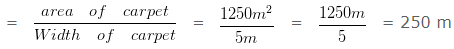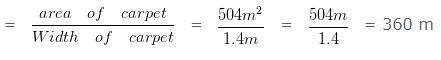# Find the cost of carpeting the room

Question 1

A room is 125 m long and 10 m broad. Find the cost of carpeting the room with a carpet of width 5 m at the rate of ₹ 20 per metre?

Explanation

Length of the room = 125 m

Breadth of the room = 10 m

Area of the floor of the room = ( 125 x 10 ) m² = 1250 m²

Area of carpet required = 1250 m²

Length of the carpet =Rate of carpeting = 20 per metre.

Cost of carpeting = ₹ ( 250 x 20 ) = ₹ 5000

Question 2

A room is 36 m long and 14 m broad. Find the cost of carpeting the room with a carpet of width 1.4 m at the rate of ₹ 12 per metre?

Explanation

Length of the room = 36 m

Breadth of the room = 14 m

Area of the floor of the room = ( 36 x 14 ) m² = 504 m²

Area of carpet required = 504 m²

Length of the carpet =Rate of carpeting = 12 per metre.

Cost of carpeting = ₹ ( 360 x 12 ) = ₹ 4320